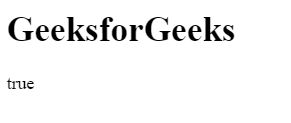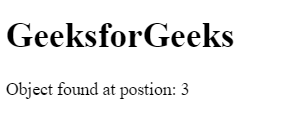# How to check if an array includes an object in JavaScript?

• Last Updated : 20 Jul, 2021

There are various methods to check an array includes an object or not.
Using includes() Method: If array contains an object/element can be determined by using includes() method. This method returns true if the array contains the object/element else return false.
Syntax:

`array.includes( element/object, startingPosition )`

Example:

## html

 ```<``html``>`` ` `<``head``>``    ``<``title``>``        ``JavaScript includes() Method``    `````` ` `<``body``>`` ` `    ``<``h1``>GeeksforGeeks``     ` `    ``<``p` `id``=``"geeks"``>`` ` `     ` `    ````    ``<``script``>``        ``var obj = {"geeks1":10, "geeks2":12}``        ``var gfg = ["geeks1", "geeks2", obj];``         ` `        ``/* Use JavaScript includes() method */``        ``var num = gfg.includes(obj, 0);``         ` `        ``document.getElementById("geeks").innerHTML``                    ``= num;``    `````` ` `        `

Output:Using some() Method: The some() method uses the function for its evaluation and it executes the function once for each element present in the array. If it finds the object/element in the array then it returns true and stop the execution for remaining elements otherwise return false.
Syntax:

`array.some( function(currValue, arrIndex, arrObj), this )`

Example:

## html

 ```<``html``>`` ` `<``head``>``    ``<``title``>``        ``JavaScript includes() Method``    `````` ` `<``body``>`` ` `    ``<``h1``>GeeksforGeeks``     ` `    ````    ``<``script``>``     ` `        ``/* Declare an array */``        ``var arr = ["geeks1", "geeks2", "geeks3",``                    ``{1:"geeks4", 2:"geeks5"}];``                     ` `        ``var boolVar = arr.some( ``            ``value => { return typeof value == "object" } );``             ` `        ``document.write(boolVar);``    `````` ` `                    `

Output:Using filter() Method: The filter() method creates the array of all those elements/objects that passes the checking condition.
Syntax:

`array.filter( function(currValue, arrIndex, arrObj), this )`

Example:

## html

 ```<``html``>`` ` `<``head``>``    ``<``title``>``        ``JavaScript includes() Method``    `````` ` `<``body``>`` ` `    ``<``h1``>GeeksforGeeks``     ` `    ``<``p` `id``=``"geeks"``>`` ` `     ` `    ````    ``<``script``>``        ``var obj = {"geeks1":10, "geeks2":12}``        ``var arr = ["geeks1", "geeks2", "geeks3", obj];``         ` `        ``if(arr.filter(value=> value==obj).length > 0)``            ``document.write("true");``        ``else``            ``document.write("false");``    `````` ` `                    `

Output:Using findIndex() Method: The findIndex() method returns the position of searched object/element if it present in the array and stop the execution for rest elements. If the element/object not found then return -1.
Syntax:

`array.findIndex( function(currValue, arrIndex, arrObj), this )`

Example:

## html

 ```<``html``>`` ` `<``head``>``    ``<``title``>``        ``JavaScript includes() Method``    `````` ` `<``body``>`` ` `    ``<``h1``>GeeksforGeeks``     ` `    ````    ``<``script``>``     ` `        ``/* Declare an array */``        ``var arr = ["geeks1", "geeks2", "geeks3",``                ``{"geeks1":10, "geeks2":12}];``                 ` `        ``var num = arr.findIndex( ``            ``value => { return typeof value == "object" } );``             ` `        ``document.write("Object found at position: " + num);``    `````` ` `                    `

Output:JavaScript is best known for web page development but it is also used in a variety of non-browser environments. You can learn JavaScript from the ground up by following this JavaScript Tutorial and JavaScript Examples.

My Personal Notes arrow_drop_up